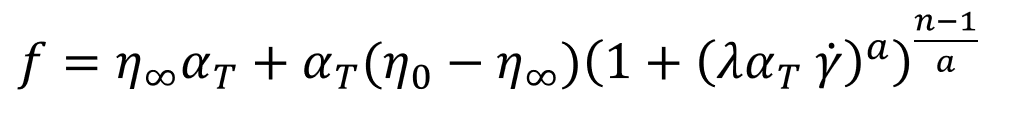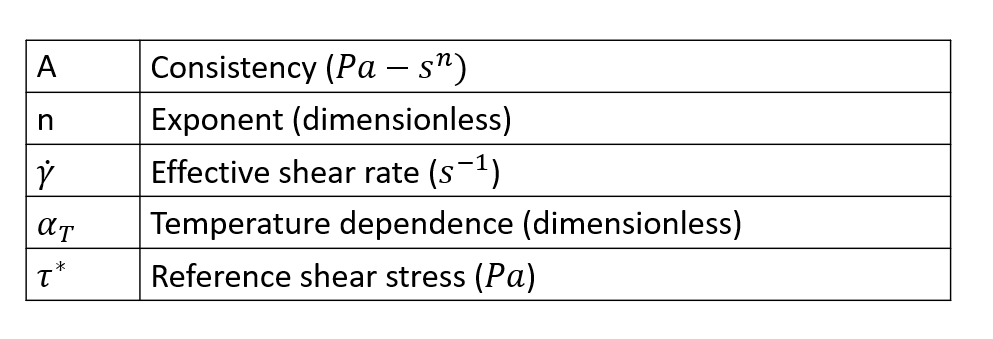# Carreau Yasuda Model

Carreau Yasuda model is expressed by the following equation. This model has five parameters, zero shear viscosity, infinite shear viscosity, time constant, transition parameter, and power law exponent.This model has a well defined upper (zero shear viscosity) and lower (infinite shear viscosity) limits for the polymer viscosity. Typically, the value of transition parameter is 2. Time constant lambda alters the nonlinear behavior of the fluid and its dependence on the shear rate. If the time constant is 0, then the fluid becomes Newtonian, with a high value for the time constant, eta becomes a strong function of shear rate. By suitable choice of parameters, many other models can be mimicked by this model.

## Syntax

 Polymer PolymerName { ConstitutiveModel = "Carreau" Density = ρ SpecificHEat = Cp (T) Conductivity = K (T) CoeffOfThermalExpansion ρ Volumetric Heat Source = Qvol TimeConstant = λ Exponent = n ZeroShearViscosisty = η0 InfiniteShearViscosity = ηinf TransitionParameter = a TemperatureDependence = "None" }
Parameter Description Units Data Type Condition Typical Value
ConstitutiveModel Describes the model used None String Required "Carreau"
Density Density of the polymer kg/m^3 Constant Required 995.0
SpeicficHeat Specific heat at constant pressure J/kg/K Constant/F(T) Required 2000.0
Conductivity Thermal conductivity W/m?k Constant/F(T) Required 0.167
CoeffOfThermalExpansion Indicates the change in volume with change in temperature 1/K Constant Required 1.0e-05
VolumetricHeatSource Heat generated/removed in the volume by methods like elctrical heating W/m63 Constant Required 0.0
Exponent Power law index, defines the dependency of viscosity on shear rate None Constant Required 0.66
ZeroShearViscosity Value of viscosity as shear rate tends to 0 Pa-s Constant Required 100.00
InfiniteShearViscosity Value of viscosity as shear rate tends to infinity Pa-s Constant Required 0.1
TransitionParameter A parameter of Carreau Yasuda model None constant Required 2
TimeConstant A parameter of Carreau Yasuda model s Constant Required 1
TemperatureDependence See temperature Dependence None string Required "WLF"
ReferenceTemeprature Temperature at which data is calculated for the initialization step. K Constant Required only if TD is not "None" 533
FreezeTemperature This is the no flow temperature. Below this temperature, material ceases to flow. K Constant Required only if TD is not "None" 350
ActivationEnergy A parameter required by Arrhenius model. J/mol Constant Required only if Td is Exp(Q/RT) 1662
UniversalGasConstant A parameter from state equation PV = nRT, R is universal gas constant J/mol/K Constant Required onlyh if TD is Exp (Q/RT) 8.314
TemperatureSensititvy A derived parameter which has the same physical meaning as Q/R. K Constant Required only if TD is Exp(Tb/T) 2000 K
WLFConstant1 Constant C1 of WLF model none Constant Required only if TD is WLF 17.44
WLFConstant2 Constant C2 of WLF model. This is like DeltaT, hence the value is same in K and Celsius. K Constant Required only if TD is WLF 51.6
GlassTransitionTemperature Temperature below with polymer molecules ceases to move (frozen). There are few definitions of this term. K Constant Required only if TD is WLF 320
Beta Parameter in the relationship Exp(-Beta(DeltaT)) None Constant Required only if TD is exp(-Beta(Delta T)) 0.005

F(T) - Function of Temperature. Can be specified as a TABLE1 or tcl function.

TD - TemperatureDependence.